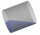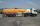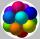Rotary cylinder 2

Base circumference of the rotary cylinder has same length as its height. What is the surface area of cylinder if its volume is 250 dm3?

Result

S =  248.64 dm2

Solution:Leave us a comment of example and its solution (i.e. if it is still somewhat unclear...):Be the first to comment!To solve this example are needed these knowledge from mathematics:

Tip: Our volume units converter will help you with converion of volume units.

Next similar examples:

1. Cylinder surface, volumeThe area of the cylinder surface and the cylinder jacket are in the ratio 3: 5. The height of the cylinder is 5 cm shorter than the radius of the base. Calculate surface area and volume of cylinder.
2. Volume and surfaceCalculate the volume and surface area of the cylinder when the cylinder height and base diameter is in a ratio of 3:4 and the area of the cylinder jacket is 24 dm2.
3. JarFrom the cylinder shaped jar after tilting spilled water so that the bottom of the jar reaches the water level accurately into half of the base. Height of jar h = 7 cm and a jar diameter D is 12 cm. How to calculate how much water remains in the jar?
4. Cylinder surface areaVolume of a cylinder whose height is equal to the radius of the base is 678.5 dm3. Calculate its surface area.
5. The volumeThe volume of a solid cylinder is 260 cm3 the cylinder is melt down into a cuboid, whose base is a square of 5cm, calculate the height of the cuboid and the surface area of the cuboid
6. Gasoline tank cylindricalWhat is the inner diameter of the tank, which is 8 m long and contains 40 cubic cubic meters of gasoline?
7. KitchenKitchen roller has a diameter 70 mm and width of 359 mm. How many square millimeters roll on one turn?
8. A cylindrical tankA cylindrical tank can hold 44 cubic meters of water. If the radius of the tank is 3.5 meters, how high is the tank?
9. Circular poolThe 3.6-meter pool has a depth of 90 cm. How many liters of water is in the pool?
10. BottleA company wants to produce a bottle whose capacity is 1.25 liters. Find the dimensions of a cylinder that will be required to produce this 1.25litres if the hight of the cylinder must be 5 times the radius.
11. Cuboid surfaceDetermine surface area of cuboid if its volume is 52.8 cm cubic and length of the two edges are 2 cm and 6 cm.
12. Cuboid - VabFind the surface of the cuboid when its volume is 52.8 cubic centimeters, and the length of its two edges is 2 centimeters and 6 centimeters.
13. Surface of cuboidFind the surface of the cuboid if its volume is 52.8 cm3 and the length of its two edges is 2 cm and 6 cm.
14. Cube 1-2-3Calculate the volume and surface area of the cube ABCDEFGH if: a) /AB/ = 4 cm b) perimeter of wall ABCD is 22 cm c) the sum of the lengths of all edges of the cube is 30 cm.
15. Spheres in sphereHow many spheres with a radius of 15 cm can fits into the larger sphere with a radius of 150 cm?
16. Cube wallsFind the volume and the surface area of the cube if the area of one of its walls is 40 cm2.
17. CuboidCuboid has a surface of 516 cm2. Side a = 6 cm and b = 12 cm. How long is the side c =?